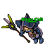## Wednesday, May 5, 2021

### TibiaGoals Riddles E8R04

Comment down below!

1.5+7=12

Pinguzz

2.respuesta 5 + 7 = 12 , se mueve una flecha del 6 para que quede en 5 y se le pasa al 1 para que se convierta en un 7
Chiki Exotic

3.respuesta : 5+7 = 12, se mueve una flecha del 6 para que quede en 5 y se la pasa al 1 para que se convierta en un 7
Wazonnick Exotic

4.5 + 7 = 12

Shizurie

5.6 - 1 ≠ 12
(?)

6.7.5+7=12, easy :D

8.you can take the arrow from the 6 and remove it on the bottom left making it a 5 and dragging it over and rotating it 90 degrees to the 1 making it a 7. Which would then complete the equation 5 plus 7 equals 12

9.Answer: 5 + 7 = 12

Char: Dyaniixz

10.11.12.13.Move the bottom left arrow to the top of the 1 make the equation 5 + 7 = 12

Xarkost Eilia

14.5 + 7 = 12
Bloody Evan

15.Hej! :) Move the lower-left arrow from the 6 and add it on top of the 1, so the equation says "5+7=12". :)

16.5 + 7 = 12
Mian Stone'arrow

17.5+7=12 Valeya Junalini

18.5 + 7 = 12; Change 6 to 5 + 1 change to 7. Procente.

19.Respuesta : El numero 6 cambia a 5 y el numero 1 a 7 dando como resultado 5 + 7 = 12

20.Nehkz
Relania

5+7=12

21.Nehkz
Relania

5+7=12

22.By moving the bottom left arrow of the number 6 to top left of the number 1 and turnig it from vertical to horizontal, so that the 6 forms a 5 instead and the 1 forms a 7. 5+7=12.

-Essiee

23.The equation true by moving only one arrow is : 5+7=12

Char name: Fyori

24.5 + 7 = 12

Marulina

25.Character - Ragge Storm

To solve this riddle u need to move the left arrow from the number 6, so it becomes number "5", and move it to the number "1" so u get the number "7". Then u have 5+7 = 12.

26.5+7=12

Lovely Olka

27.5+7=12 - Mikolaj

28.5+7=12
Eternal hunger
Antica

29.5+7=12
Eternal hunger
Antica

30.5+7=12
Eternal hunger
Antica

31.5+7=12.... Bebitha consentida

32.5+7=12.... Bebitha consentida

33.5+7=12.... Bebitha consentida

34.Zuali
https://gyazo.com/210f4a804b42ed58db568e1fbe2eb0d3
5+7=12

35.36.Move the left vertical arrow off of 6, making it 5.
Place the arrow horizontally by the top of 1, making it a 7.
5 + 7 = 12

Character: Elee Bae

37.5+7 =12
Sir wuachachucho

38.5+7=12
Vysterion

39.5+7=12

Pameliin

40.Myo Disturbed

By moving the left bottom arrow from the six to the one at the right and put it above to make it 5+7 = 12

41.R= 5 + 7 = 12
samuray de carro :)

42.5 + 7 = 12
Character: Amy Meow

43.5+7= 12
Moviendo el inferior izquierdo y formando el 7
By Aurora Ventresca

44.45.5+7 = 12

Skyelie Hasulhoff

46.5 + 7 =12

move the bottom left and add to the 1 on top

Toxic Hasulhoff

47.Congratulations to all of you!

People who won't qualify to Event #9 lottery:
- Ardyjan (1st, 3rd, 4th riddle missing)
- Dune Elvath (2nd, 3rd, 4th riddle missing)
- Mikasza Ackerman (2nd, 3rd, 4th riddle missing)
- Mistery paladin (1st, 2nd, 4th riddle missing)
- Quack (2nd, 3rd, 4th riddle missing)
- Soulcalibur (2nd, 3rd, 4th riddle missing)